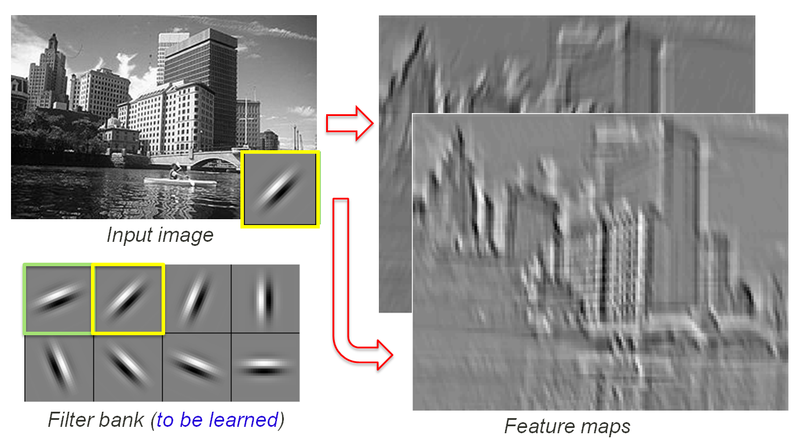2 views

I am currently seeing the API of theano,

theano.tensor.nnet.conv2d(input, filters, input_shape=None, filter_shape=None, border_mode='valid', subsample=(1, 1), filter_flip=True, image_shape=None, **kwargs)

where the filter_shape is a tuple of (num_filter, num_channel, height, width), I am confusing about this because isn't that the number of filters decided by the stride while sliding the filter window on the image? How can I specify on the filter number just like this? It would be reasonable to me if it is calculated by the parameter stride (if there is any).

Also, I am confused with the term feature map as well, is it the neurons at each layer? How about the batch size? How are they correlated?

by (33.1k points)

In neural networks, there are some layers to perform computations. Each layer consists of a specific number of neurons. So the number of filters in CNN is the number of neurons present in a neural net. Each neuron performs a different convolution The number of filters is the number of neurons

There is a feature map in neural nets, which is the result of applying a filter and its size is a result of the window size of your filter and stride.

The following image explains the concept of CNN at depth level:You can notice that there are two convolutional filters, that are applied to the input image, resulting in two different feature maps.

Each pixel in the image represents the feature map as an output of the convolutional layer.

Let’s say if you are using 28x28 input images and a convolutional layer with 20 7x7 filters and stride 1, you will get 20 22x22 pixels to feature maps at the output of this layer. You can use the same representation to train your CNN on RGB images.